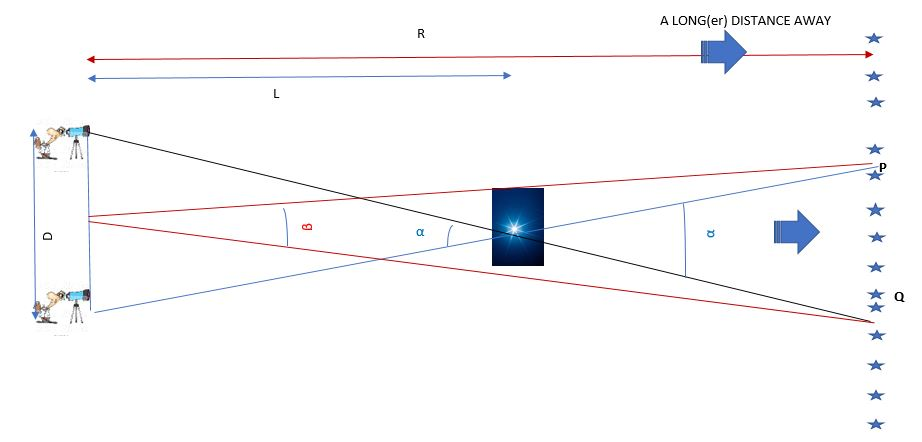# Cosmology: Distance Measurements – Parallax (Post #3)This post describes the cool methods people use to figure out how far away stars and galaxies are. Figuring out how far away your friend lives is easy – you walk or drive at a constant speed in a straight line from your home to their house – then once you know how much time this took, you multiply speed times the time of travel to get the distance to your friend’s house.

This might seem like an excessively detailed description of a simple task, but don’t forget that the ancients would have difficulty with several things here – how do you travel at a constant speed and how do you measure time of travel? The first seems like a possible task, but how do you measure time ? Humans have invented many ways to measure time – water clocks (reported in Greece, China and India), sand clocks, burning knotted ropes. The Antikythera mechanism, if confirmed to be an astronomical device, would be similar to a true mechanical clock, but it took the ability to work metal and the Industrial Revolution to reliably mass-produce clocks.

This was the most effective way to measure distances for many years; just travel there and keep notes!

The heavenly object closest to us appears to be the moon. Very early, to some extent by Aristarchus, but really by Edmund Halley (whose comet is more famous than he is), it was realized that Parallax could be used to figure distance to far away objects, without actually traveling there. Parallax is illustrated below – its the perceived angular shift in an object’s position relative to far-away things when you shift your viewing position. You experience this all the time when you see nearby things shift when you look from one eye, then the other.The diagram above is a little busy, so let me explain it.$L$ is the distance that we are trying to measure, between the Earth (where the fellow with the telescope is) and the bright blue star.$R$ is the distance to the starry background, that is${\bf really}$ far away. Since$R$ is${\bf much}$ bigger than$L$,  you should be able to convince yourself that the angles$\alpha$ and$\beta$ are very close to each other. From basic geometry, to a good approximation$D = \alpha L$

which means$L = \frac {D}{\alpha}$. We just need to compute$\alpha$, but it is roughly equal to$\beta$.$\beta$ is the just the angular separation of the stars$P$ and$Q$, which you could measure with, for instance, a sextant.

We know$D$, which is the baseline of the measurement. If you use your two eyes, it is a few inches. You could get ambitious and make measurements in summer and winter, when the baseline would be the diameter of the Earth’s orbit (OK, the orbit is very nearly a circle). The result is that you can figure out how far away the bright blue star is by computing the perceived angular shift.

The farther away something is, the smaller the perceived angular shift. For a long time, people could not measure angular shifts for really distant objects and made the assumption that the method was wrong for some reason, for they couldn’t believe stars could be that far away.

The state of the art in the parallax measurement was the Hipparcos satellite and is currently the Gaia satellite (as well as Hubble). Distances upto 30,000 light years are capable of being measured. For reference, we think Andromeda galaxy is 2.5 million light years away and the Milky Way’s dark matter halo extends out to 180,000 light years. So to measure out to these distances needs different techniques, which will be discussed in the next post.

1.abhirar says:
1.Simply Curious says: